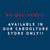\$9.50 Postage! Fast Flat Rate - Order \$75-\$150 and pay just \$5.00 Delivery - Order over \$150 and Receive Free Shipping *Conditions Apply# Aquarium Volume Calculator

When you’re buying a new fish tank it is important to know how many litres your tank can hold, so that you be accurate when adding or dosing items like medications, de-chlorinators, or knowing how many fish your tank can safely hold.

However, calculating fish tank volume can be tricky at the best of times, but with The Tech Den's aquarium volume calculator, working out the volume of a fish tank has never been easier. Our aquarium calculator uses 7 popular aquarium shapes, from cubes and rectangles, to cylinders and bow front tanks.

Instructions

• Select the shape of your aquarium
• Enter the tank dimensions
• The aquarium calculator finds the volume of your tank

• Remember that the calculations shown here are merely estimations and the how much water you should use depends on several factors such as the thickness of the glass in your tank, the objects in your tank such as sand, gravel, filters, plants, and fish.

## How to manually calculate aquarium tank sizes

If you want to calculate the aquarium size manually, you can use the formulas for volume depending on tank shape:

• How to calculate the volume of a rectangular aquarium

To calculate the volume of a rectangular aquarium simply times the height by the width by the length:

`rectangular = height * width * length`

• How to calculate the volume of a cube shaped aquarium

To calculate the volume of a cube aquarium you can use the same formula for rectangle, or simply times the length by 3:

`cube = length³ (or) length x length x length`

• How to calculate the volume of a cylinder shaped aquarium

To calculate the volume of water in a cylindrical aquarium you can use the below formula:

`cylinder = π * (diameter / 2)² * height`

If you need the volume for a half cylinder, simply use the above formula and divide by 2.

`half cylinder = π * (diameter / 2)² * height / 2`

And if you need a quarter cylinder use the below formula.

`quarter_cylinder = π * radius² * height / 4`

• Bow front and bow front corner aquarium

Bow front aquariums are a bit trickier to calculate. You need the height, width, length and a full width of the aquarium.

`bowfront = height * width * length + 0.5 * r² * (α - sin(α)) * height`

where `α` is a central angle of the circular segment, in radians and `r` is a radius of a circle.
α may be calculated as `α = 2π - 4β`, where β is an angle expressed as `tan(β) = 0.5 * length / (full_width - width)`.

For the aquarium with bow front intended to stand in the corner, the formula is:

`corner_bowfront = (0.5 * r² * (α - sin(α)) * height) / 2`